In part 1 of this series, we examined our data before building any models. Among the discoveries were missing values in some of our columns.

Missing values are an inevitable part of data analysis. Whether it's due to a faulty sensor, human error, or simply the absence of information, missing values can wreak havoc on your analysis if not handled properly. Fortunately, SAS Viya 4.0 offers a powerful toolset for imputing missing values: the dataPreprocess action set.
In this article, we'll explore the dataPreprocess action set in SAS Viya 4.0 and show you how to use it to impute missing values in your data.

# What is the definition of Missing Values?

SAS defines a missing value as a value where no data is stored for the variable in the current observation. SAS includes three types of missing values: numeric (represented by a period), character (represented by a blank space), and special numeric.

For more information on working with missing values in SAS, check out this documentation.

# What is the dataPreprocess Action Set?

At its core, the DataPreprocess Action Set allows users to transform and manipulate their data in a variety of ways. This can include tasks such as cleaning up messy or inconsistent data, and creating new variables based on existing ones.

One of the key benefits of using the dataPreprocess action set is that it can save you time and effort compared to manually performing these tasks. For example, instead of writing complex code to create a new variable with specific criteria, you can use one of the many built-in functions within the action set to do it for you, which is what we are going to do to impute missing values for the missing columns or variables.

# What imputation methods are available for the Impute Action?

The Impute action includes the following methods for interval variables:

• MAX – replaces with the maximum value
• MEAN – replaces with the mean
• MEDIAN – replaces with the median
• MIDRANGE – replaces with the mean of the maximum and minimum values
• MIN – replaces with the minimum value
• RANDOM – replaces with a uniform random value between the minimum and maximum values
• VALUE – replaces with a constant value

The Impute action includes the following methods for nominal variables:

• MODE – replaces with the mode
• VALUE – replaces with a constant value

# Let’s Impute the Missing Values in our Data

First check to see what variables have missing values.

```nmiss = conn.simple.distinct( table = HomeEquity) nmiss```

Here we can see that several of our variables have missing values with NMISS greater than zero.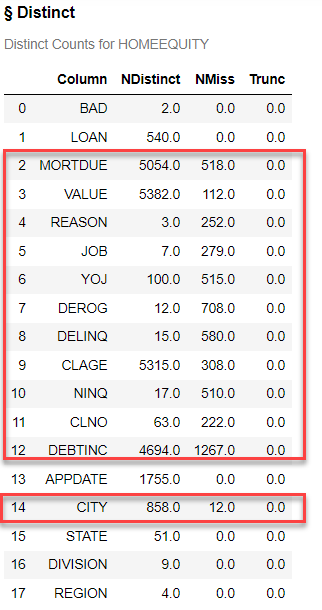Visualizing data is a crucial step in data analysis. To accomplish this, we can create a Python data frame that includes the variable name and the corresponding number of missing values.

```# Create a casDataFrame containing number of missing values for each variable tbl = HomeEquity.distinct()['Distinct'][['Column', 'NMiss']]```

By utilizing matplotlib, we can then generate a plot to depict the percentage of missing values for each variable. This practice not only enhances the overall quality of our analysis but also provides valuable insights into the data.

```# Plot the percent of missing values locally from matplotlib import pyplot as plt nr = HomeEquity.shape tbl['PctMiss'] = tbl['NMiss']/nr MissPlot = tbl.plot(x='Column', y='PctMiss', kind='bar', figsize=(8,8), fontsize=15) MissPlot.set_xlabel('Variable', fontsize=15) MissPlot.set_ylabel('Percent Missing', fontsize=15) MissPlot.legend_.remove() plt.show()```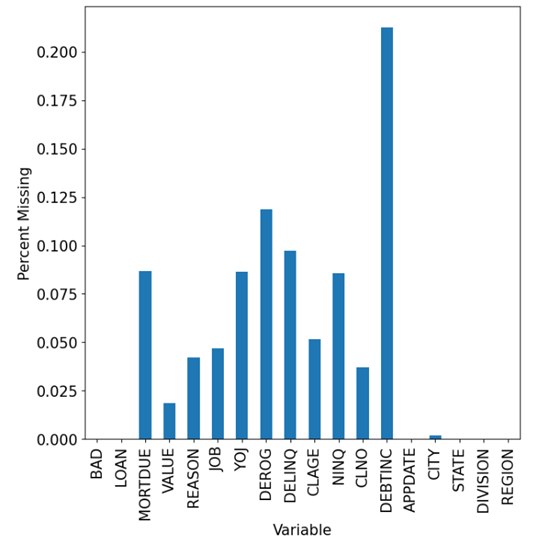Next use the dataPreprocess action set with the impute action to impute the missing values. For interval use the MEDIAN option and for nominal use the MODE option.

```conn.dataPreprocess.impute( table = 'HomeEquity', methodInterval = 'MEDIAN', methodNominal = 'MODE', inputs = list(HomeEquity)[1:], copyAllVars = True, casOut = dict(name = 'HomeEquity2', replace = True) )```

The last column two columns in the output show you the value imputed for each of the columns with missing data. For example, LOAN has a median equal to 16,300 and REASON has a MODE of DebtCon. These are the values used to replace the missing for these variables.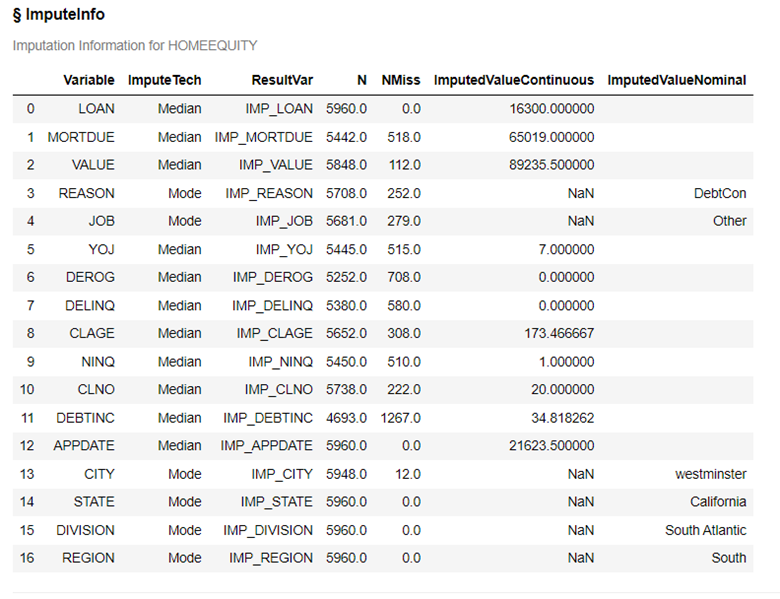A new output table is created as well.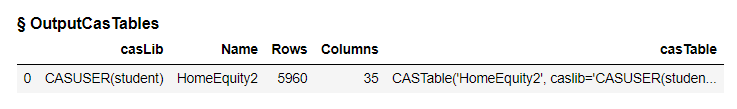Let’s check the new output table to see if we still have missing values.

```HomeEquity2 = conn.CASTable(name = "HomeEquity2") HomeEquity2.table.columnInfo()```

Notice our original columns are still in the table but new columns have been added with a prefix of IMP_ for each column and these columns contain the original values plus the imputed values in place of the missing.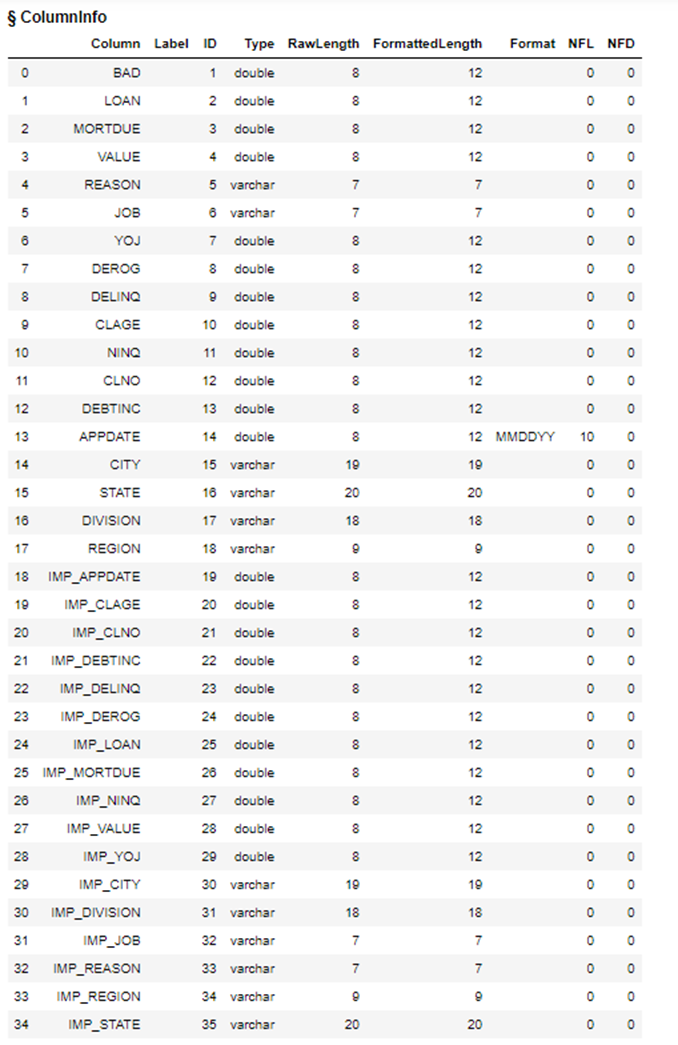To check for missing values, use the summary action.

```conn.simple.summary(table='HomeEquity2', subSet=["N","NMISS","MEAN","MIN","MAX"])```

Looking at NMISS for all of the IMP_ variables we see the value of zero, which means we have no missing values for these columns.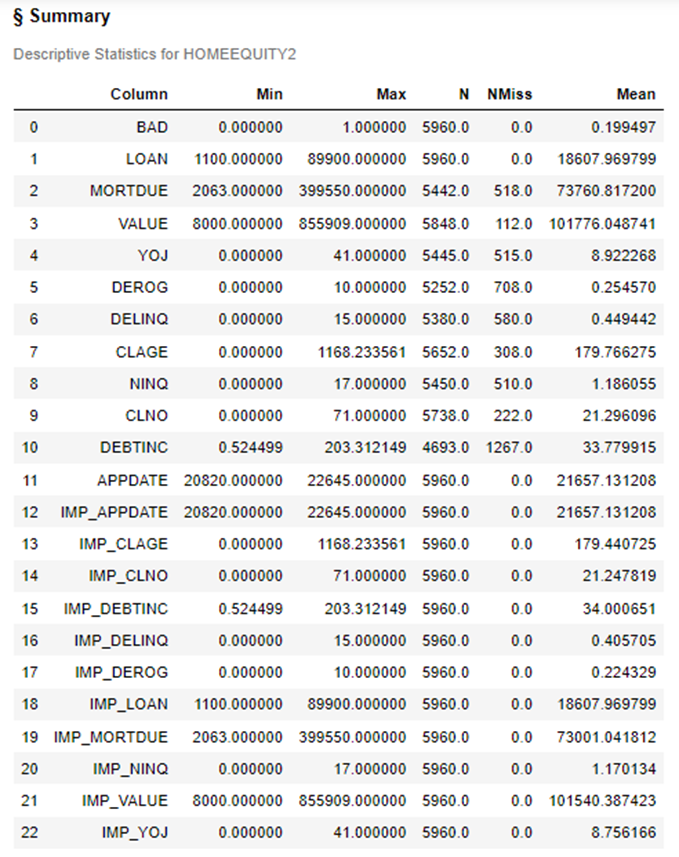# The Wrap-Up: Imputing Missing Values

Using the dataPreprocess action set with the Impute action we can easily impute our missing values to prepare our data for modeling. For additional examples for working with missing values please check out my colleague's blog post Python Integration to SAS Viya Part 21 – Impute Missing Values.

# Related Resources

Share